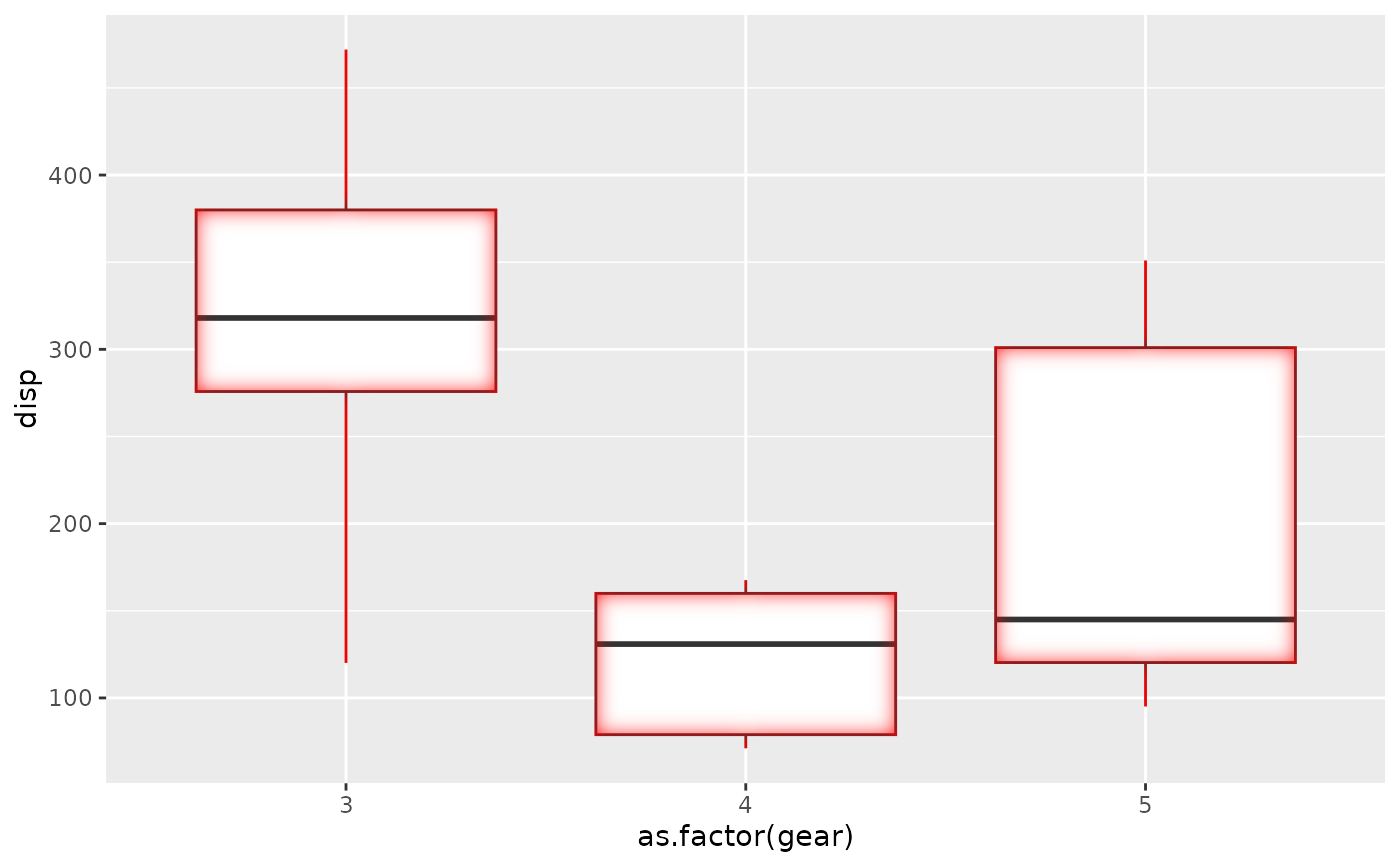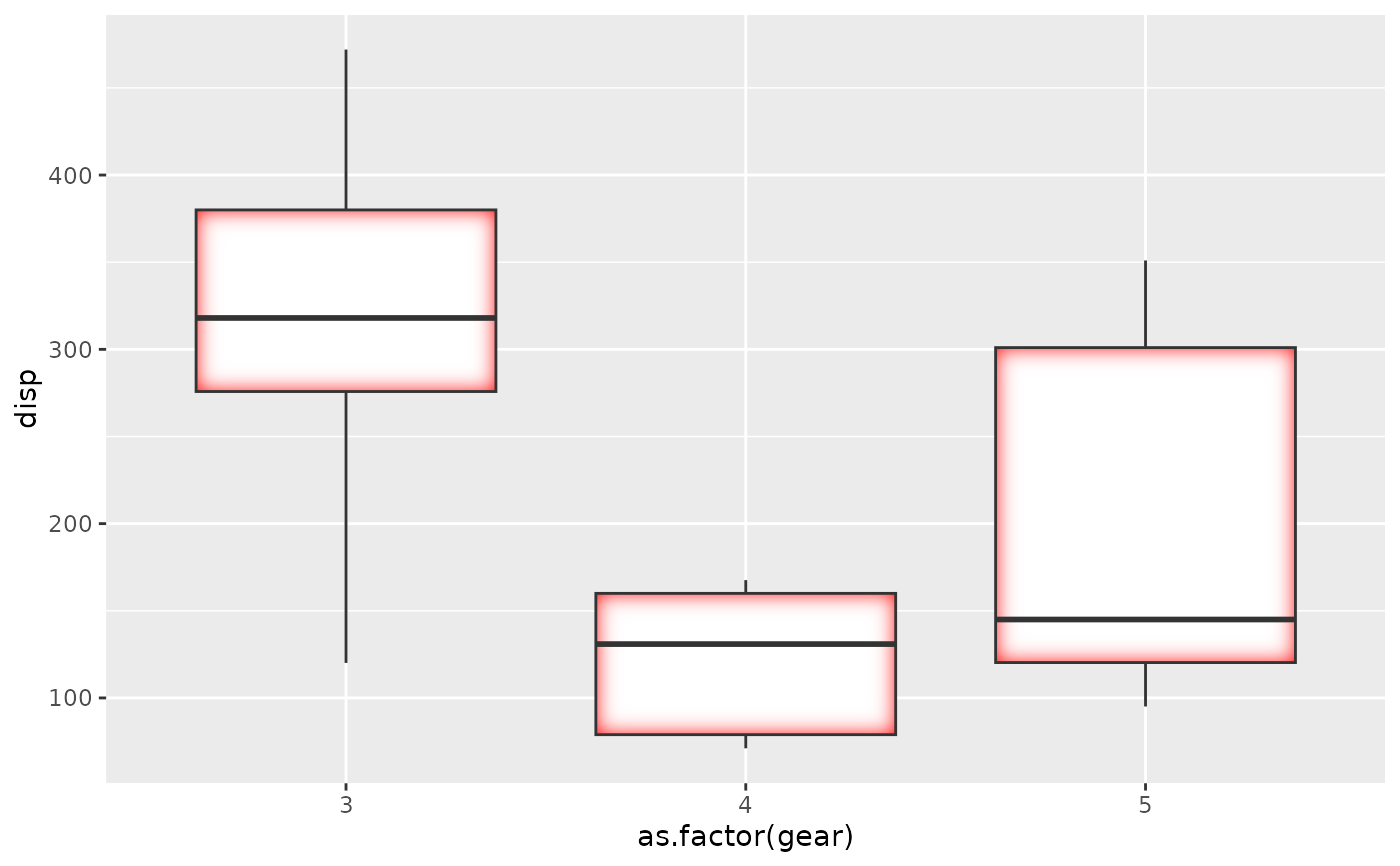This filter adds an inner glow to your layer with a specific colour and size. The best effect is often had by drawing the stroke separately so the glow is only applied to the fill.

with_inner_glow(x, colour = "black", sigma = 3, expand = 0, ...)

## Arguments

x

A ggplot2 layer object, a ggplot, a grob, or a character string naming a filter

colour

The colour of the glow

sigma

The standard deviation of the gaussian kernel. Increase it to apply more blurring. If a numeric it will be interpreted as given in pixels. If a unit object it will automatically be converted to pixels at rendering time

expand

An added dilation to the glow mask before blurring it

...

Arguments to be passed on to methods. See the documentation of supported object for a description of object specific arguments.

## Value

Depending on the input, either a grob, Layer, list of Layers, guide, or element object. Assume the output can be used in the same context as the input.

Other glow filters: with_outer_glow()

## Examples

library(ggplot2)

ggplot(mtcars, aes(as.factor(gear), disp)) +
with_inner_glow(
geom_boxplot(),
colour = 'red',
sigma = 10
)# This gives a red tone to the lines as well which may not be desirable
# This can be fixed by drawing fill and stroke separately
ggplot(mtcars, aes(as.factor(gear), disp)) +
with_inner_glow(
geom_boxplot(colour = NA),
colour = 'red',
sigma = 10
) +
geom_boxplot(fill = NA)